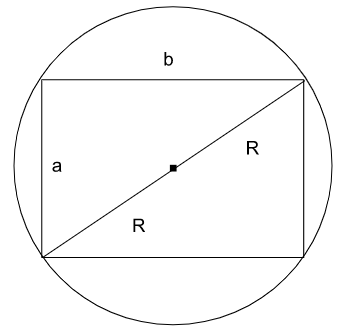# CAT 2022 Question Paper | Quant Slot 1

###### CAT Previous Year Paper | CAT Quant Questions | Question 19

CAT 2022 Quant was dominated by Arithmetic followed by Algebra. In Arithmetic, the questions were dominated by topics like Speed-time-distance, Mixture and Alligations. This year, there was a surprise. The questions from Geometry were relatively on the lower side as compared to the previous years. There were 8 TITA Qs this year. Overall this section was at a medium level of difficulty.

Question 19 : All the vertices of a rectangle lie on a circle of radius $R$. If the perimeter of the rectangle is $P$, then the area of the rectangle is

1. $$frac{P^2}{2}-2 P R$ 2. $$frac{P^2}{8}-2 R^2$ 3. $\frac{P^2}{16}-R^2$ 4. $\frac{P^2}{8}-\frac{R^2}{2}$ ## 🎉Get Upto ₹10,000 off on our CAT 23/24 courses.🎉Get flat ₹6,000 off on IPM courses.Valid till 30th September 2023! #### ★Register Now! ### Video Explanation ## Best CAT Coaching in Chennai #### CAT Coaching in Chennai - CAT 2022Limited Seats Available - Register Now! ### Explanatory AnswerLet the dimensions of the rectangle inscribed in the circle be a and b. We are being asked to express the Area of the rectangle in terms of Perimeter and Radius. Area of the rectangle = a * b Perimeter, P = 2$a + b$
a + b = $$frac { P } { 2 }$ $$ a + b ) ^ { 2 } = $frac { P ^ { 2 } } { 4 }$ $a ^ { 2 } + b ^ { 2 } + 2 a b = $frac { P ^ { 2 } } { 4 }$ Since the vertices of the rectangle subtend right angle on the circle, $a ^ { 2 } + b ^ { 2 } =$ 2 R$ ^ { 2 } = 4 R ^ { 2 }$
$a ^ { 2 } + b ^ { 2 } + 2 a b = $frac { P ^ { 2 } } { 4 }$ $4 R ^ { 2 } + 2 a b = $frac { P ^ { 2 } } { 4 }$ $a b = \frac { P ^ { 2 } } { 8 } - 2 R ^ { 2 }$ Area of the rectangle = ab = $\frac { p ^ { 2 } } { 8 } - 2 R ^ { 2 }$ The question is " All the vertices of a rectangle lie on a circle of radius $R$. If the perimeter of the rectangle is $P$, then the area of the rectangle is " ##### Hence, the answer is '$\frac{P^2}{8}-2 R^2$' Choice B is the correct answer. ###### 🎉Get Upto ₹10,000 off on our CAT 23/24 courses.🎉Flat ₹6000 off on IPM courses. ###### Prepare for CAT 2023 with 2IIM's Daily Preparation Schedule ###### Know all about CAT Exam Syllabus and what to expect in CAT ###### Already have an Account? ###### CAT Coaching in ChennaiCAT 2023 Classroom Batches Starting Now! @Gopalapuram ###### Best CAT Coaching in Chennai Introductory offer of 5000/- Attend a Demo Class ###### Best Indore IPM & Rohtak IPM CoachingSignup and sample 9 full classes for free. Register now! ##### Where is 2IIM located? 2IIM Online CAT Coaching A Fermat Education Initiative, 58/16, Indira Gandhi Street, Kaveri Rangan Nagar, Saligramam, Chennai 600 093 ##### How to reach 2IIM? Mobile:$91$ 99626 48484 / 94459 38484
WhatsApp: WhatsApp Now
Email: info@2iim.com